# MSBSHSE Solutions For SSC (Class 10) Maths Part 1 Chapter 4 Financial Planning

MSBSHSE Solutions For SSC (Class 10) Maths Part 1 Chapter 4 Financial Planning is useful for students as it helps them to score well in the class exams. We, in our aim to help students, have devised detailed chapter-wise solutions for them to understand the concepts easily. The Maharashtra Board Solutions contain detailed steps explaining all the problems that come under Chapter 4 “Financial Planning” of the Part 1 Class 10 Maharashtra Board Maths Textbook. We have followed the latest Syllabus while creating these solutions and it is framed in accordance with the exam pattern of the Maharashtra Board.

These solutions are designed by subject matter experts who have assembled model questions covering all the exercise questions from the textbook. By solving questions from the Maharashtra Board Solutions for Class 10, students will be able to clear all their concepts about “Financial Planning”. In this chapter, students will learn about GST and tax. Some of the important topics of this chapter are goods and service tax, tax invoice, tax invoice of goods purchase, tax invoice of services provided, GST in a trading chain, input tax credit, features of GST, types of taxes under GST, shares, stock Exchange. The chapter also covers a comparison of FV and MV, Rate of Return – RoR, brokerage, and taxes on share trading, GST on Brokerage Services, Mutual Fund – MF, Systematic Investment Plan, and Benefits of Mutual Funds.

## Download the PDF of Maharashtra Solutions For SSC Maths Part 1 Chapter 4 Financial Planning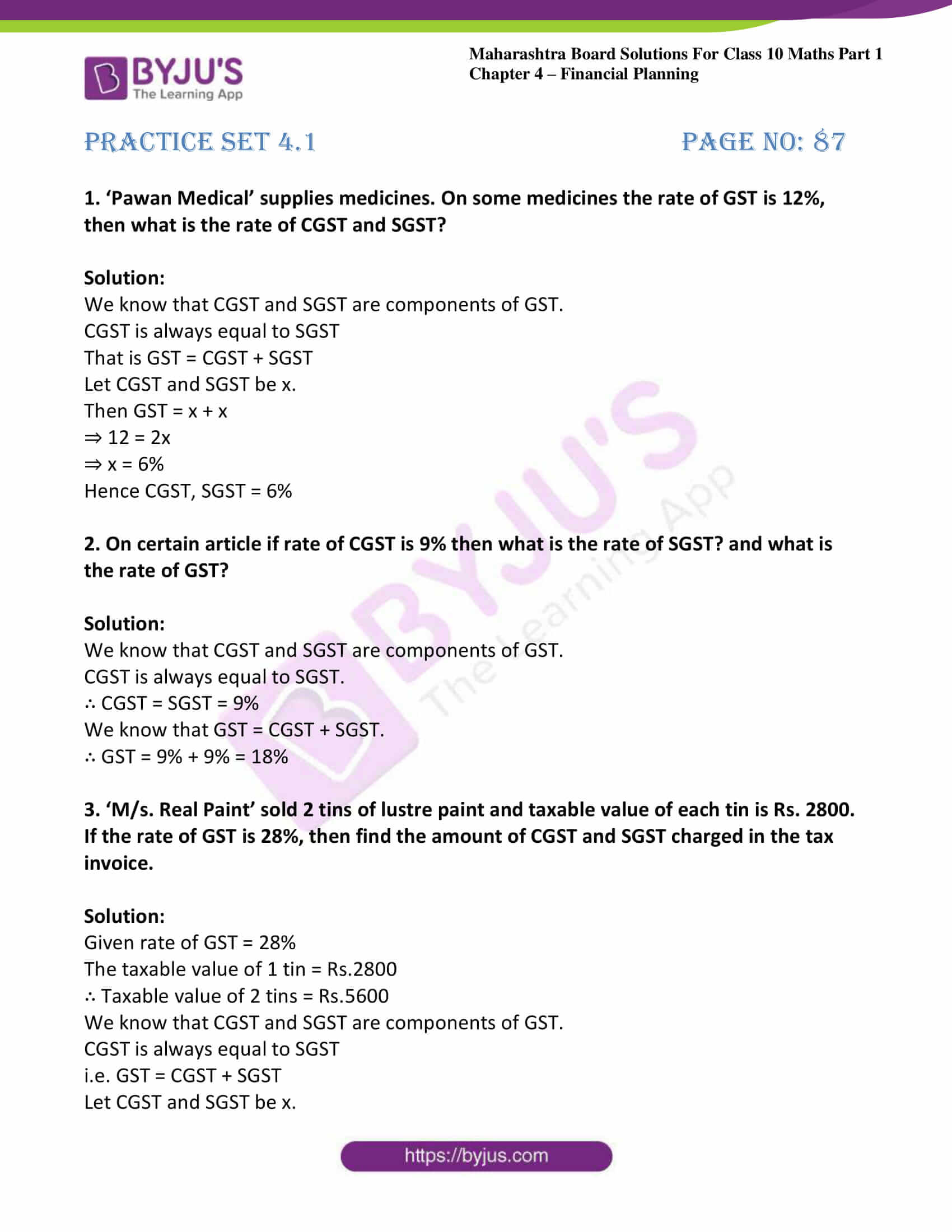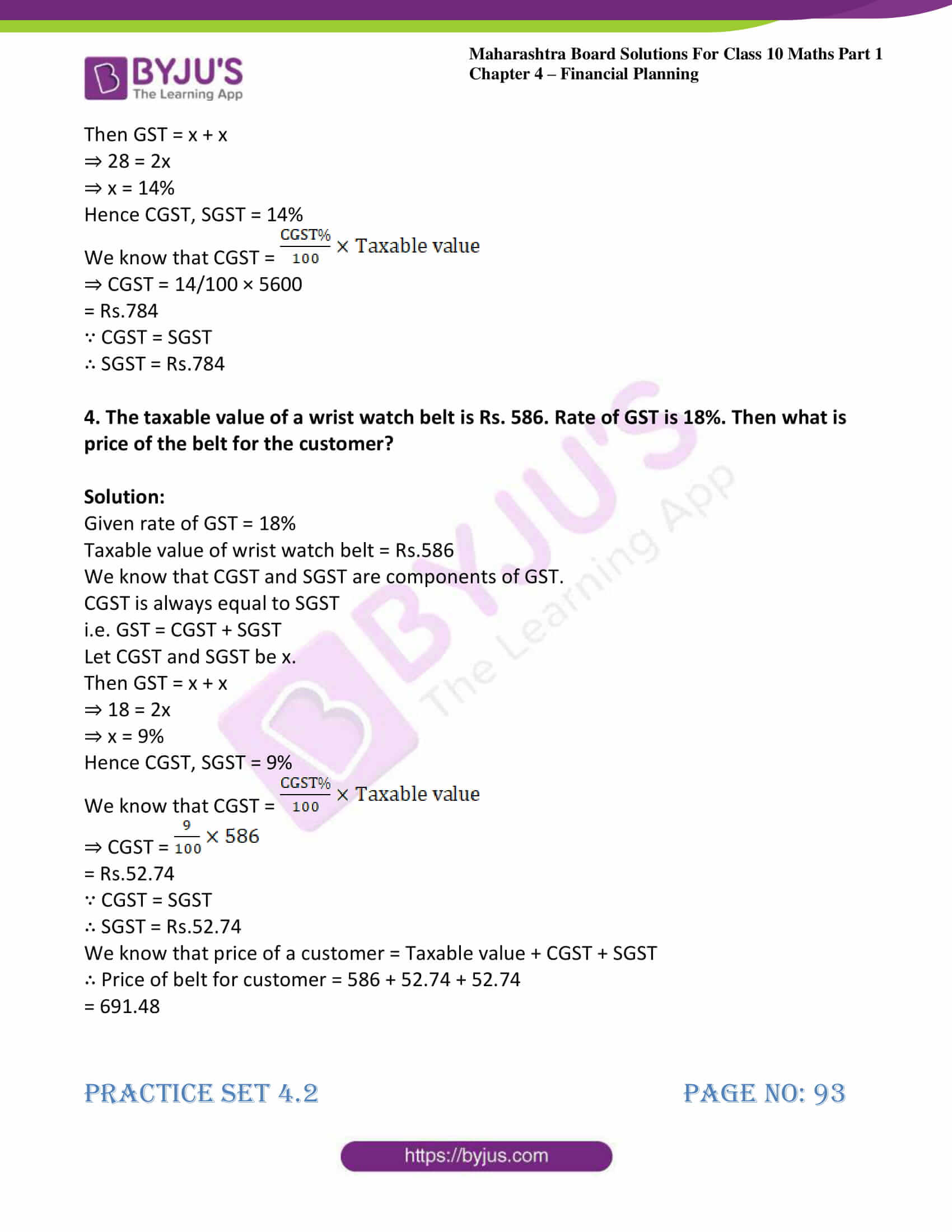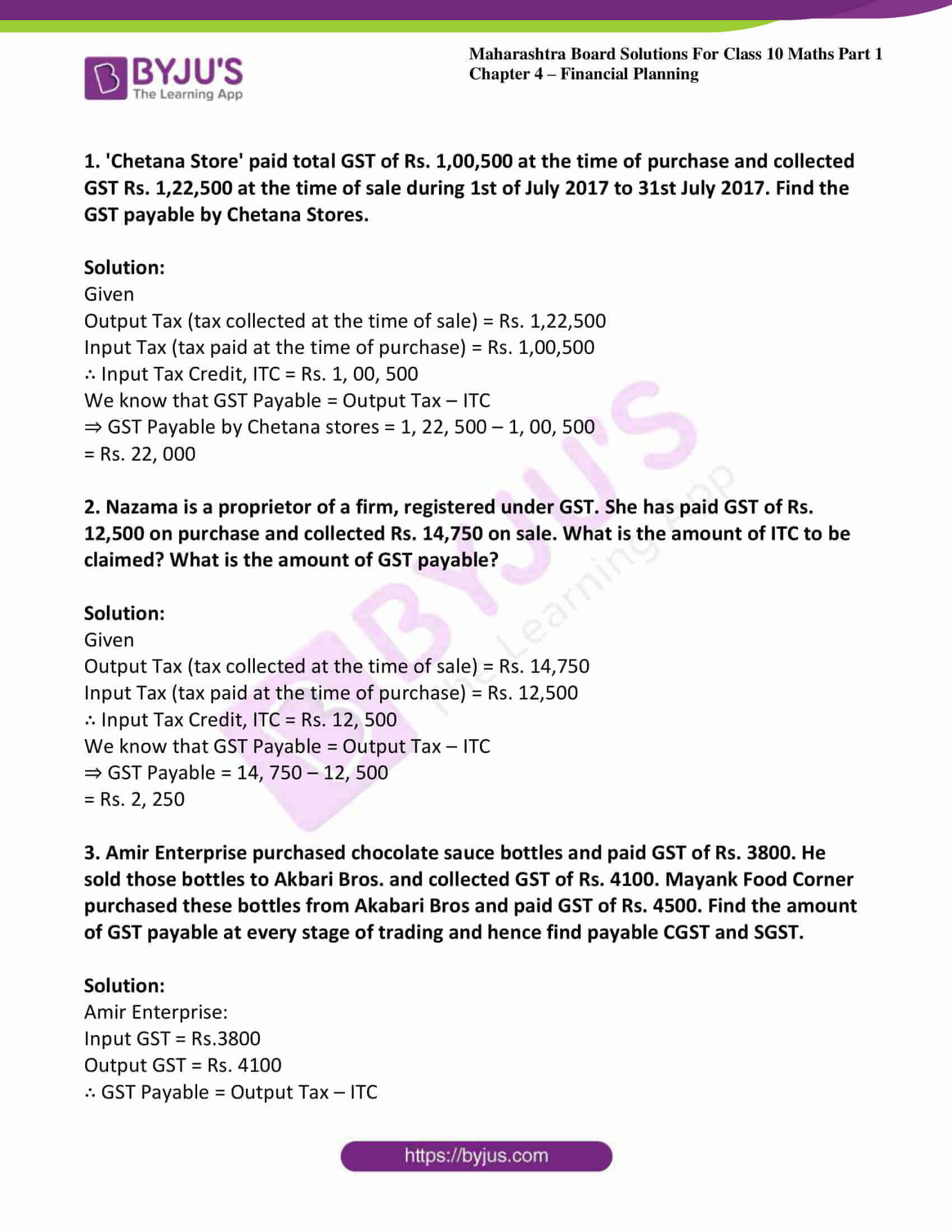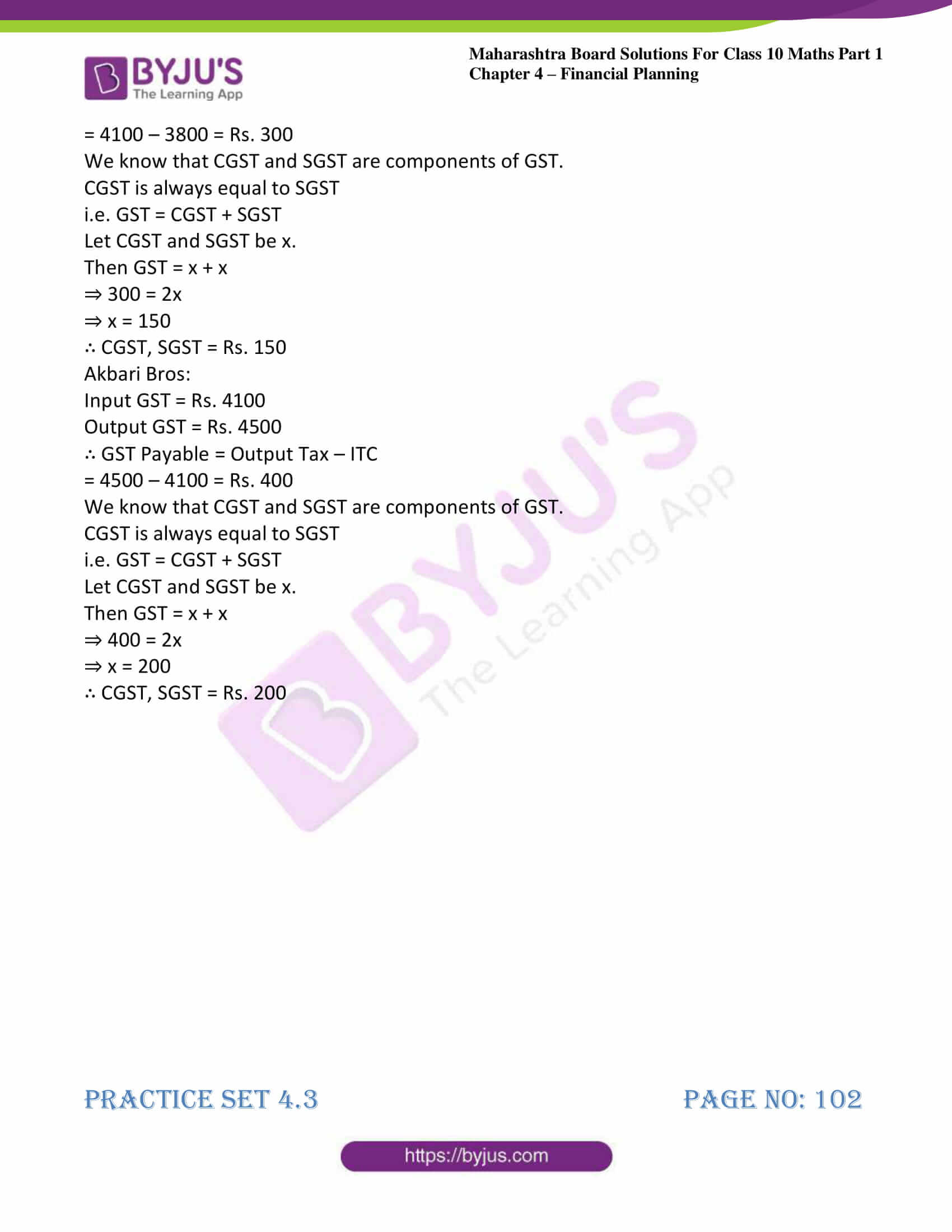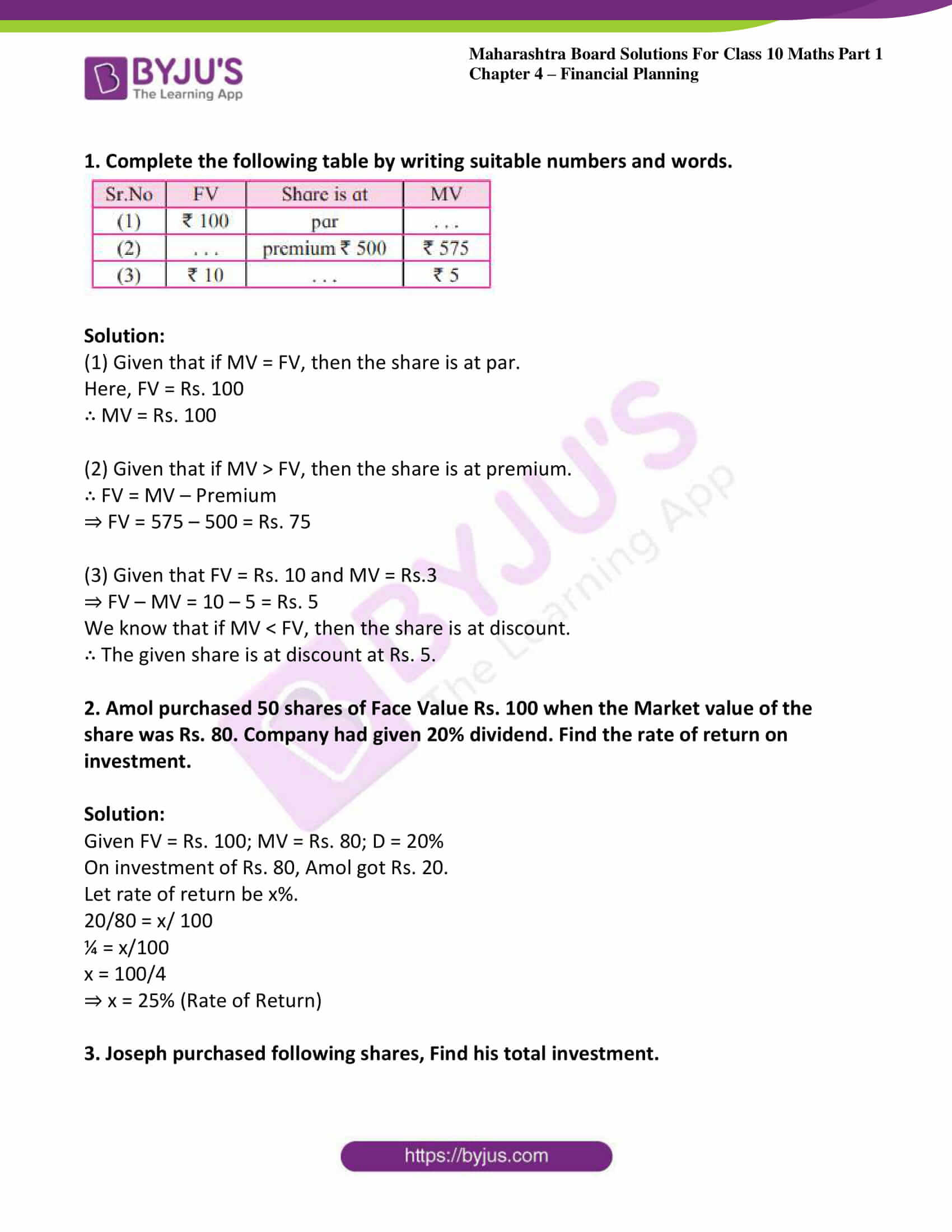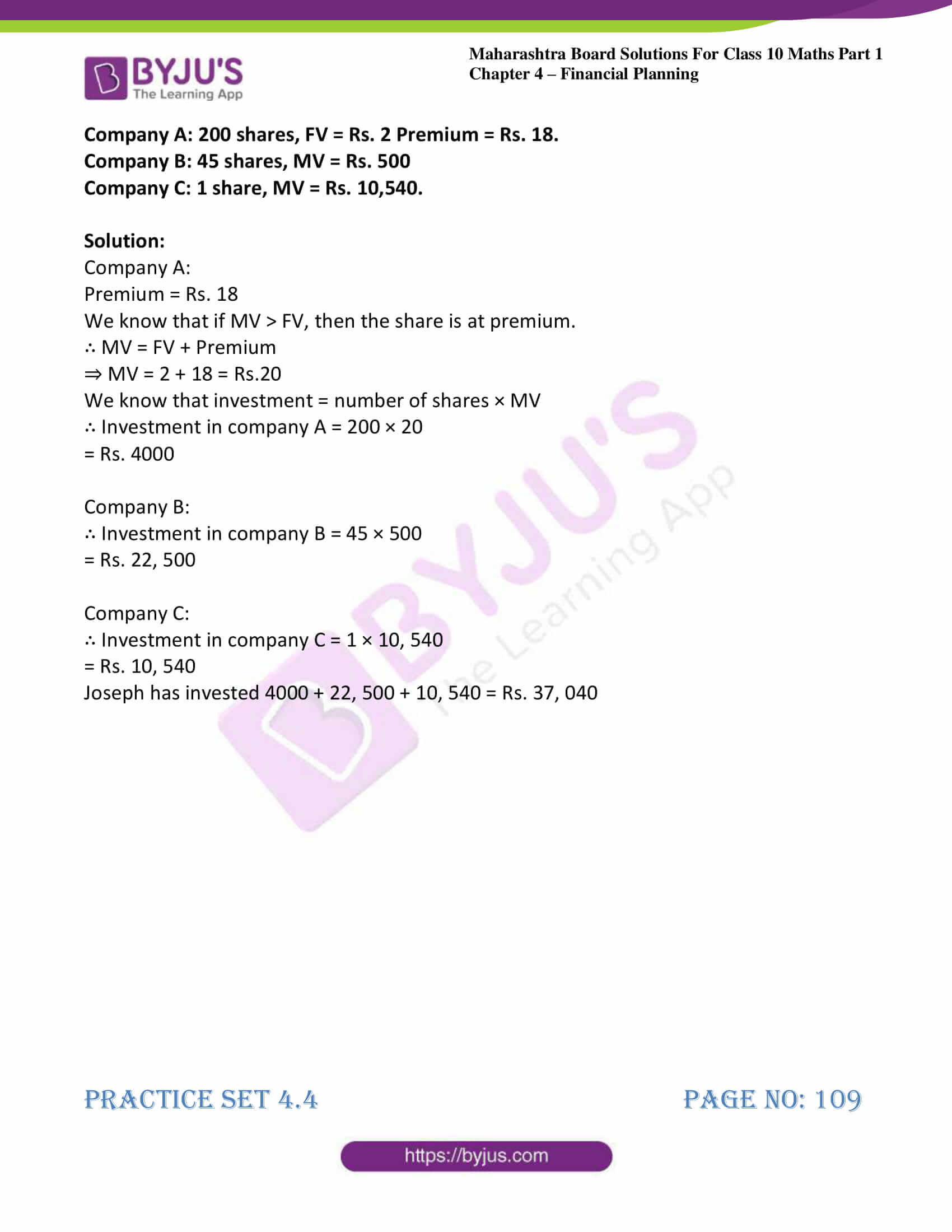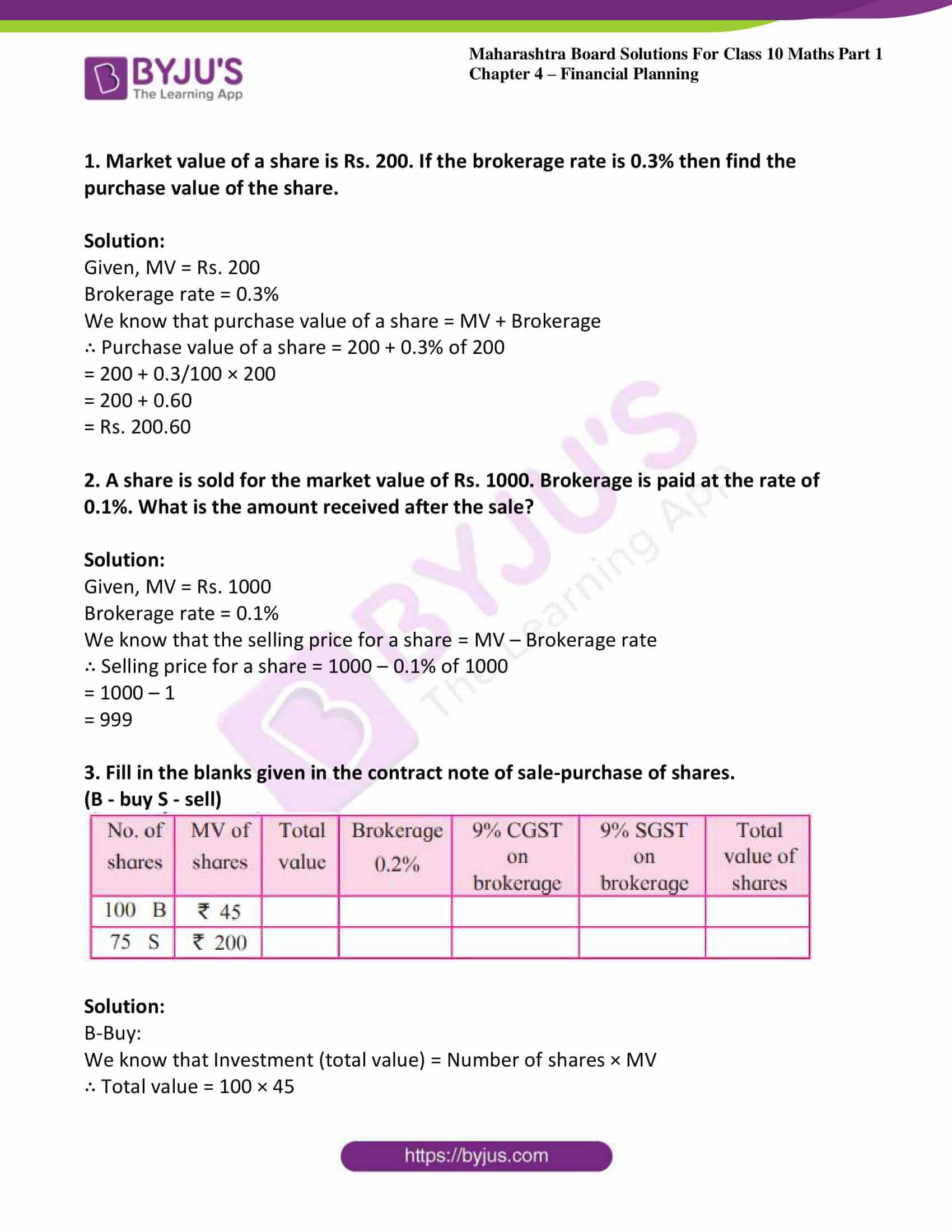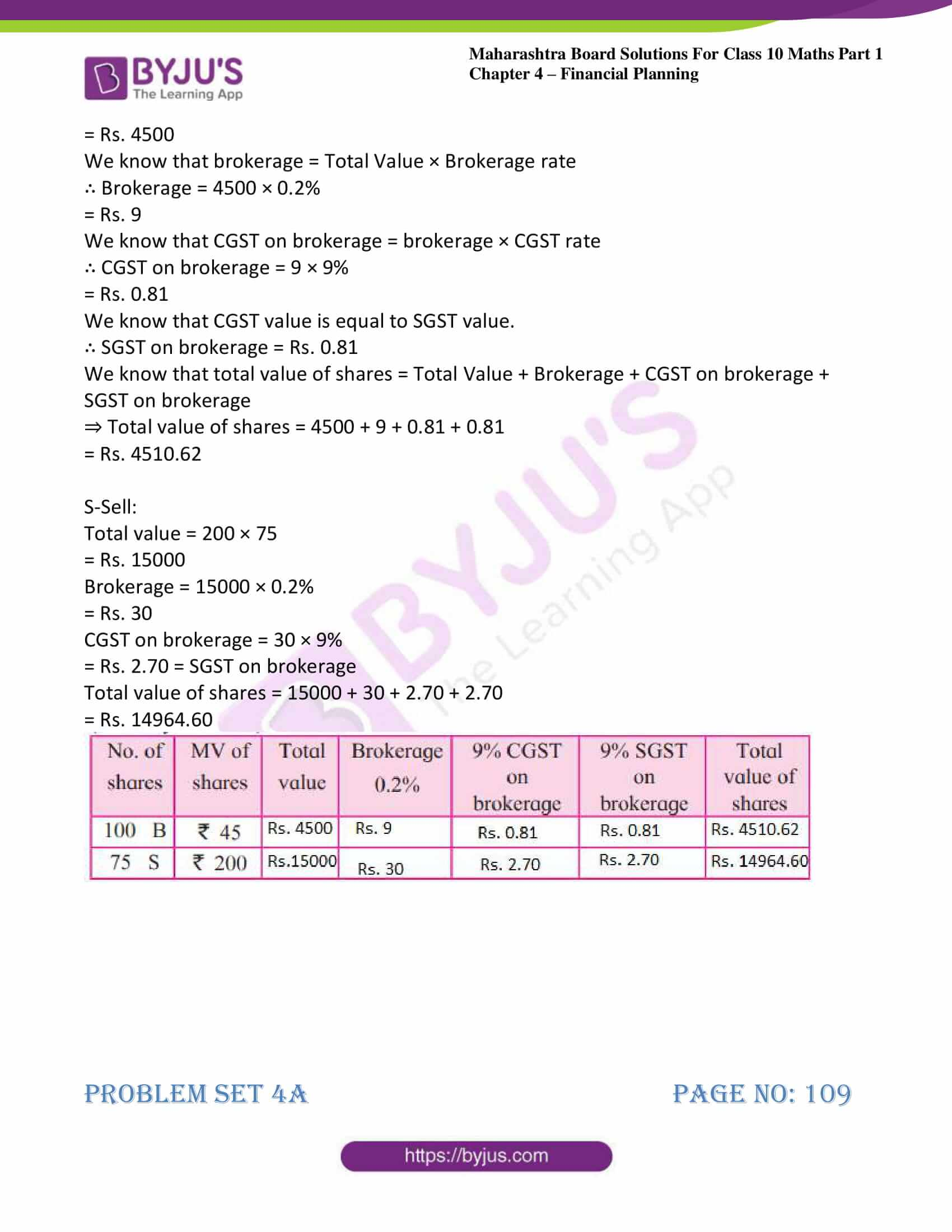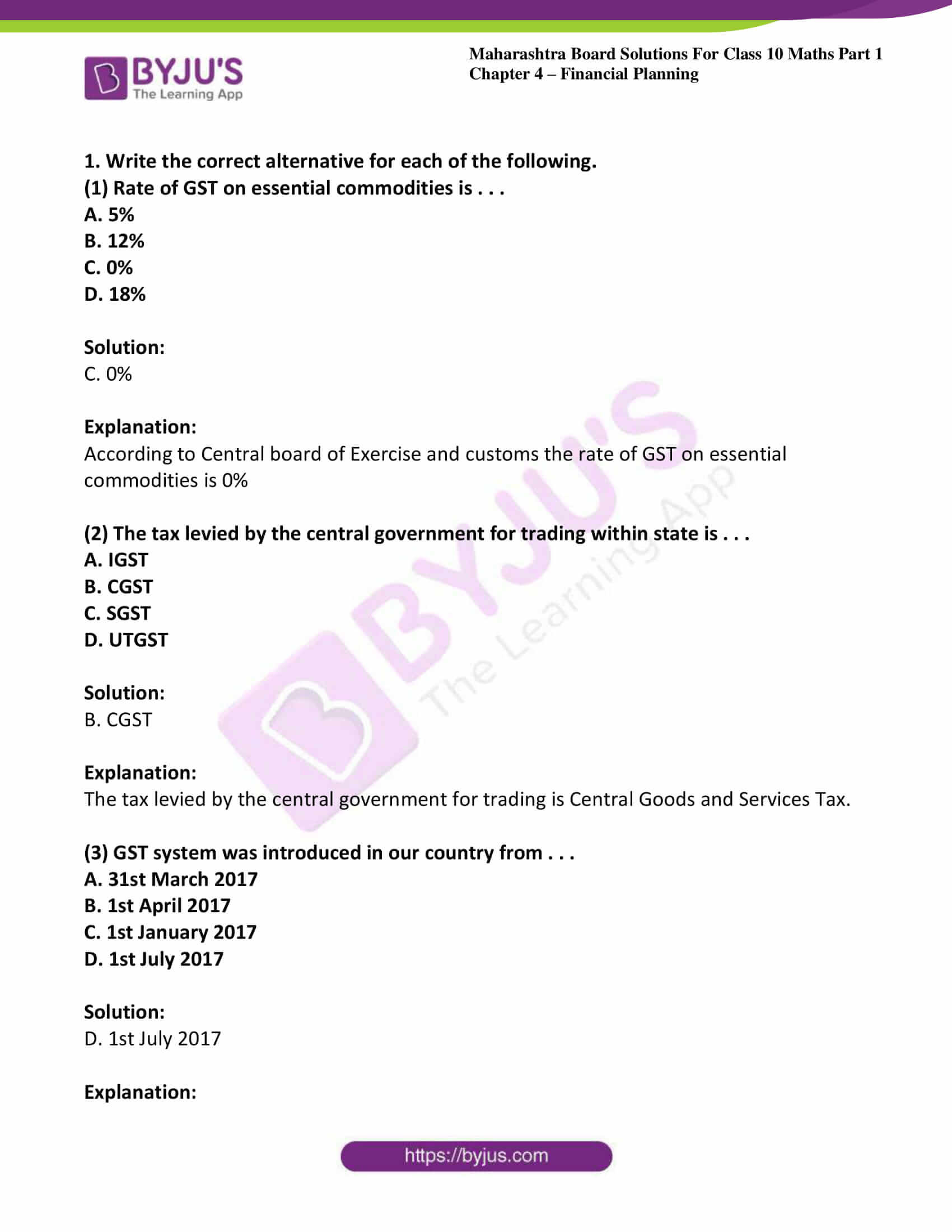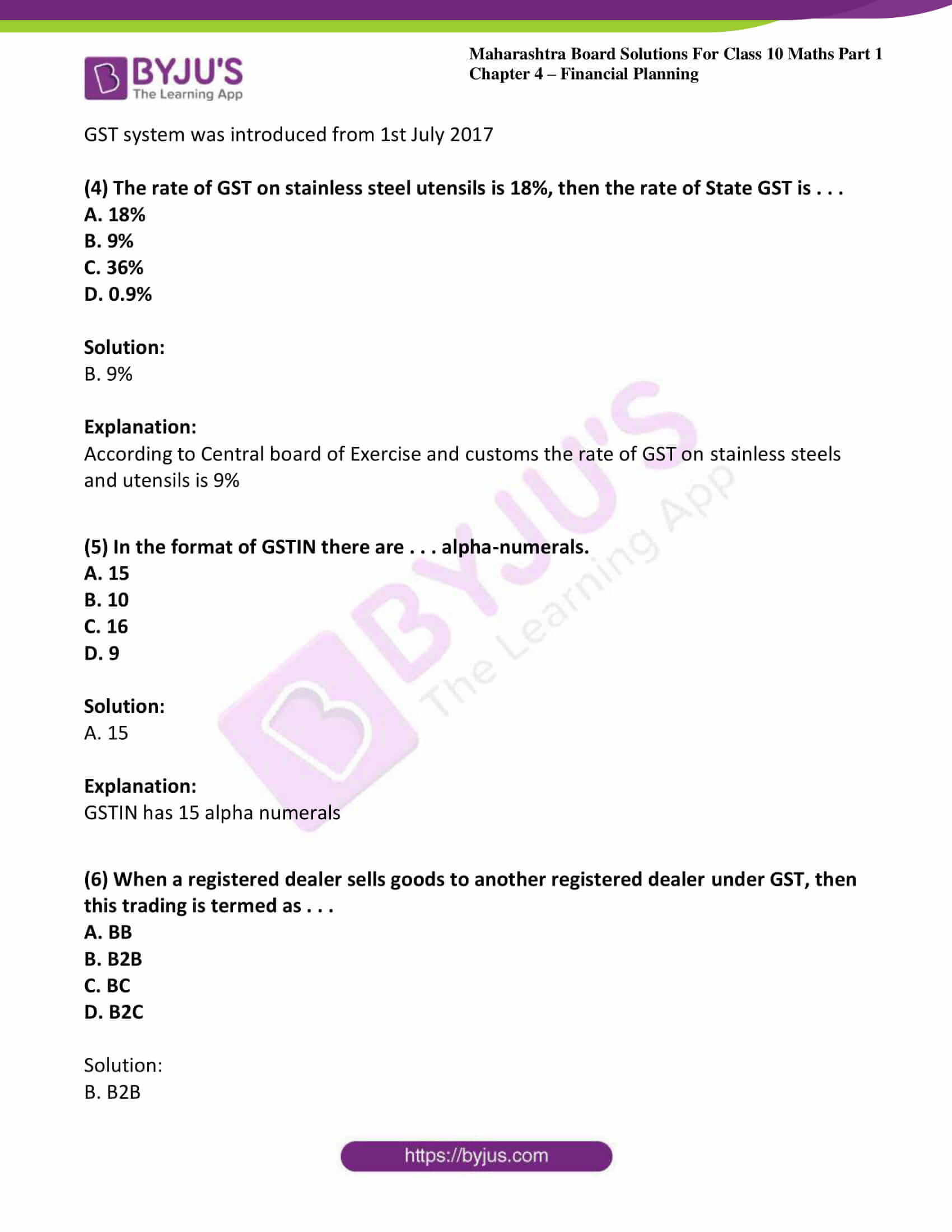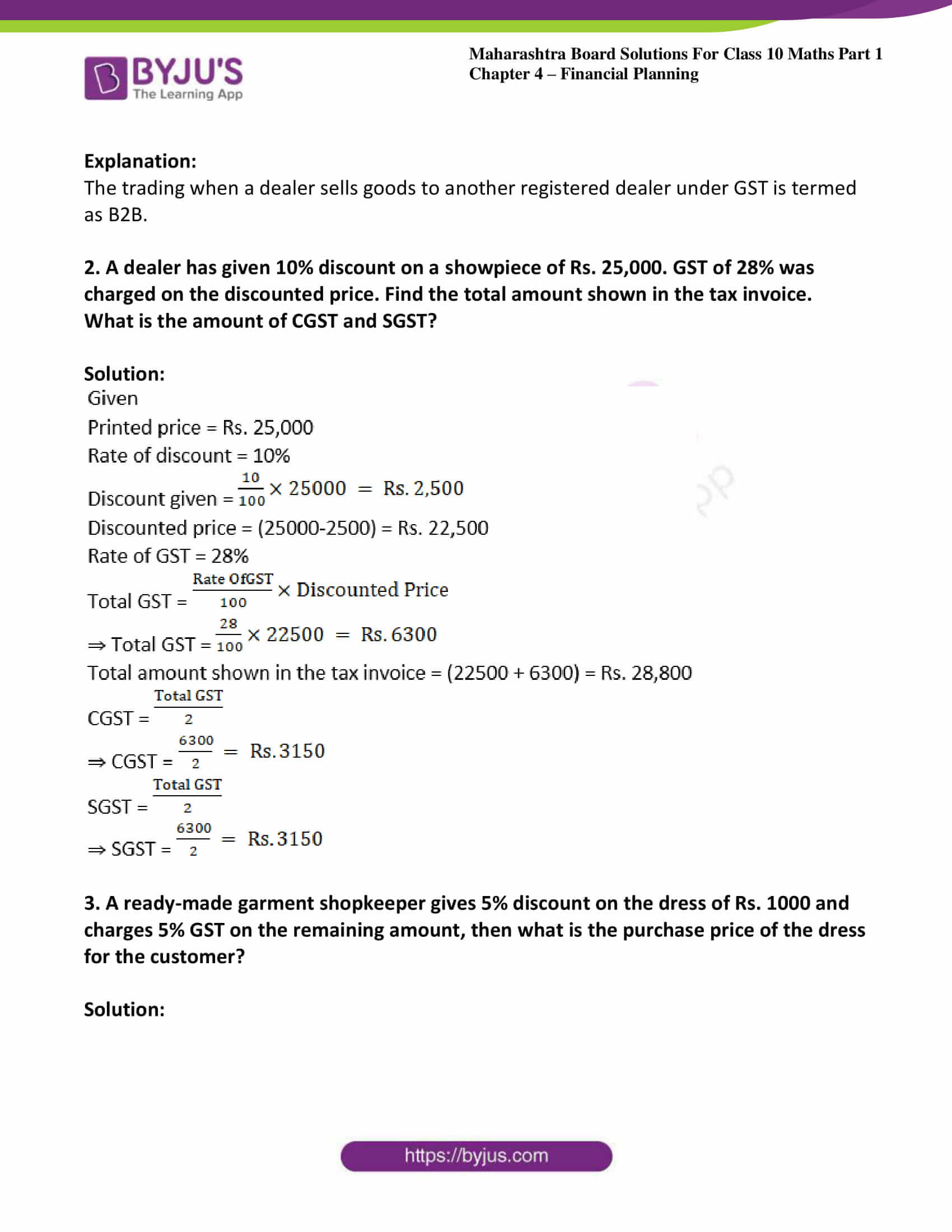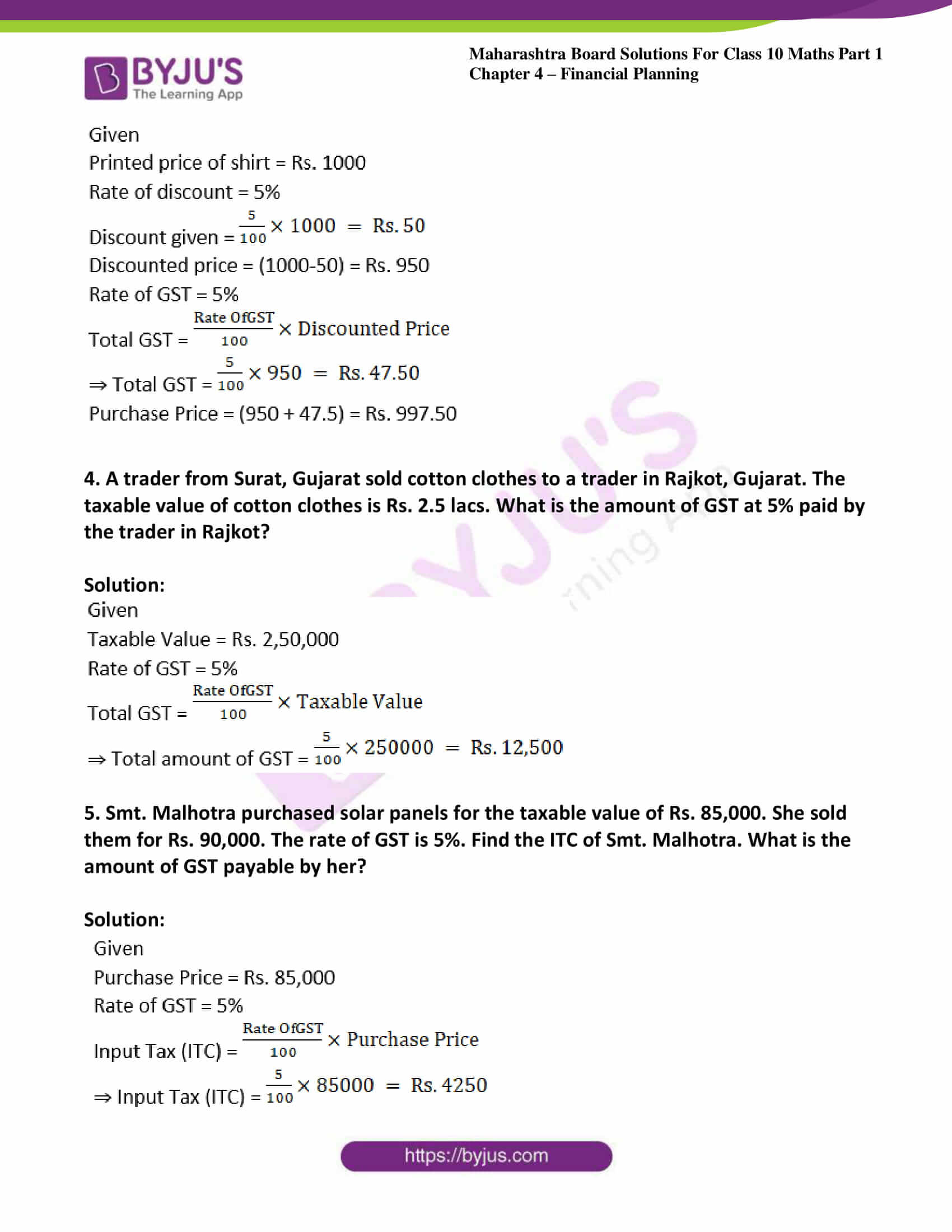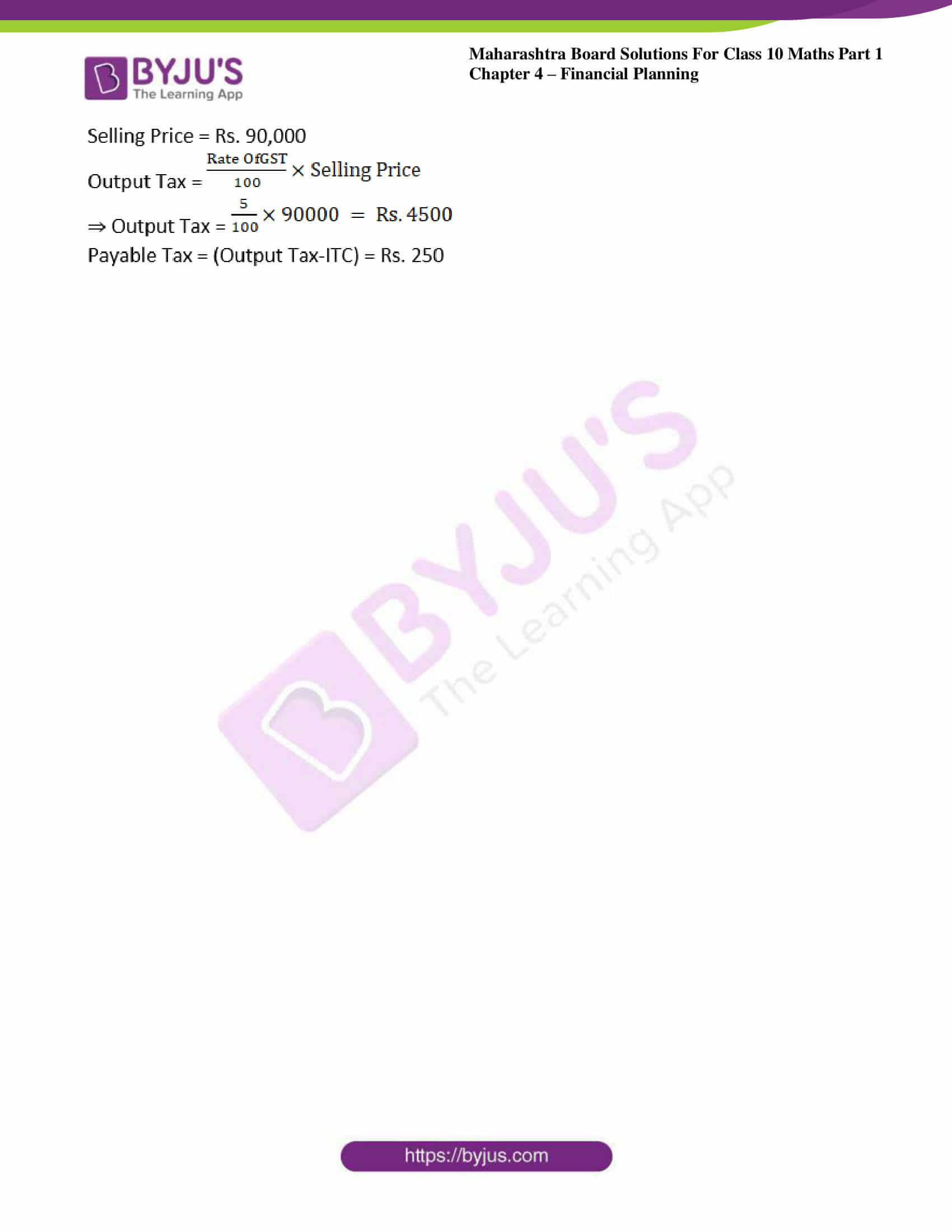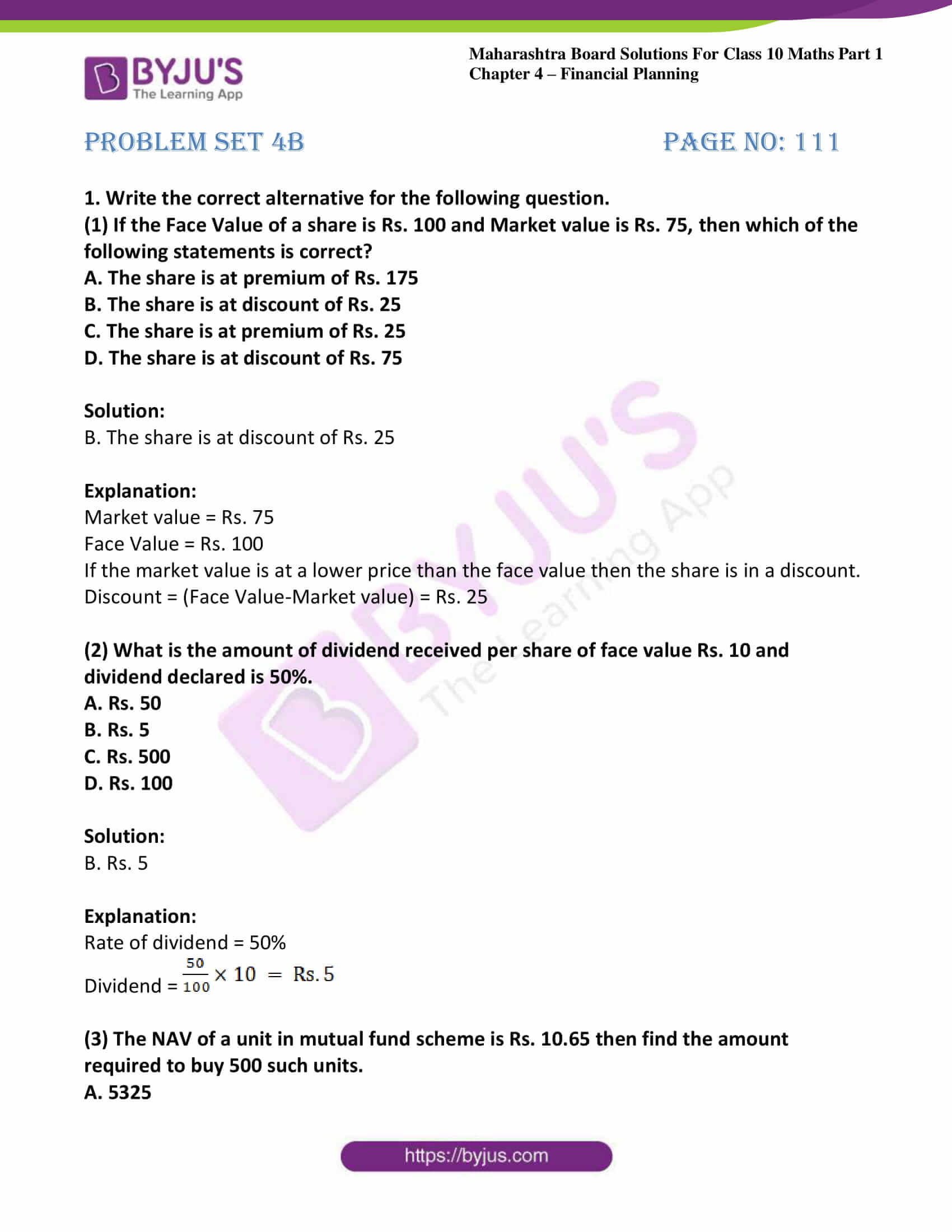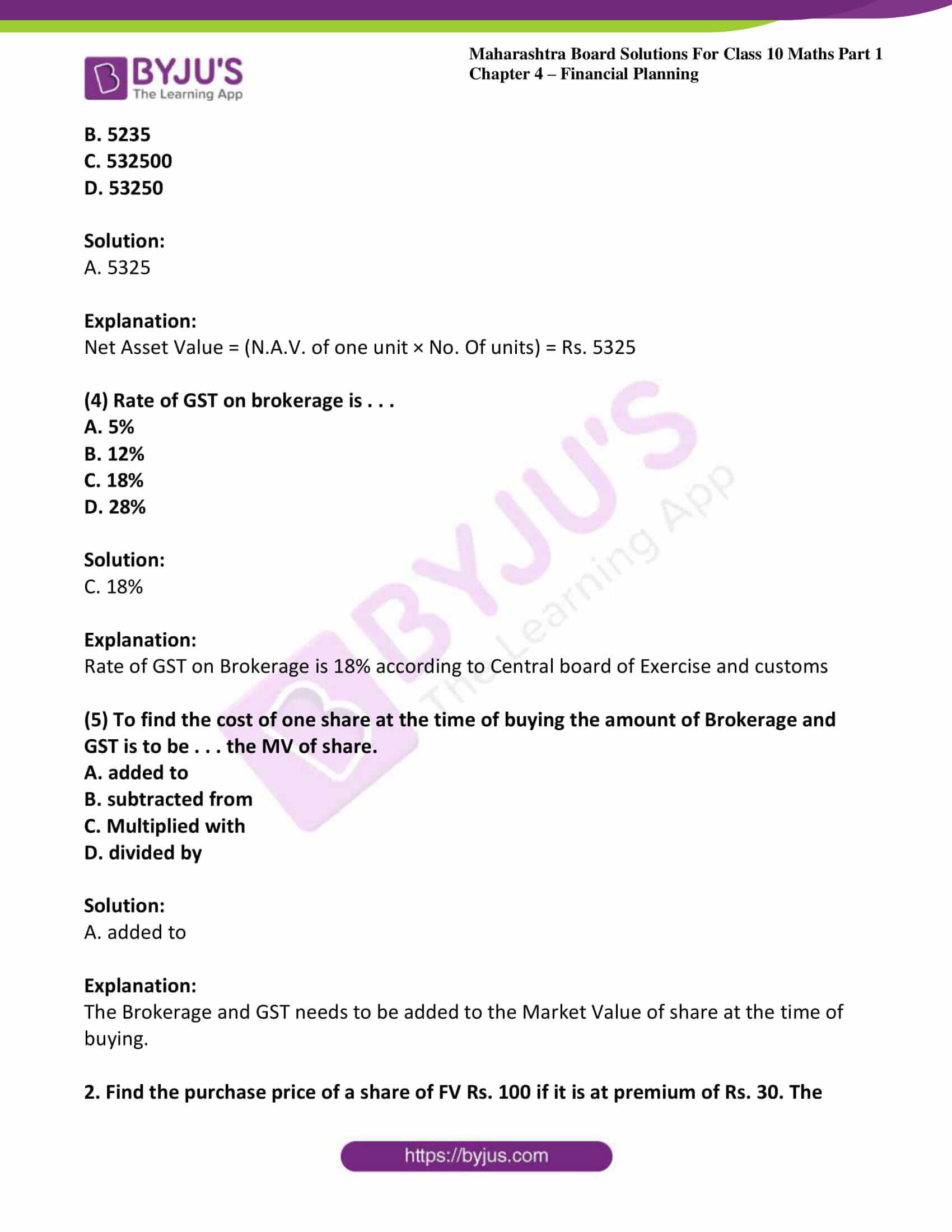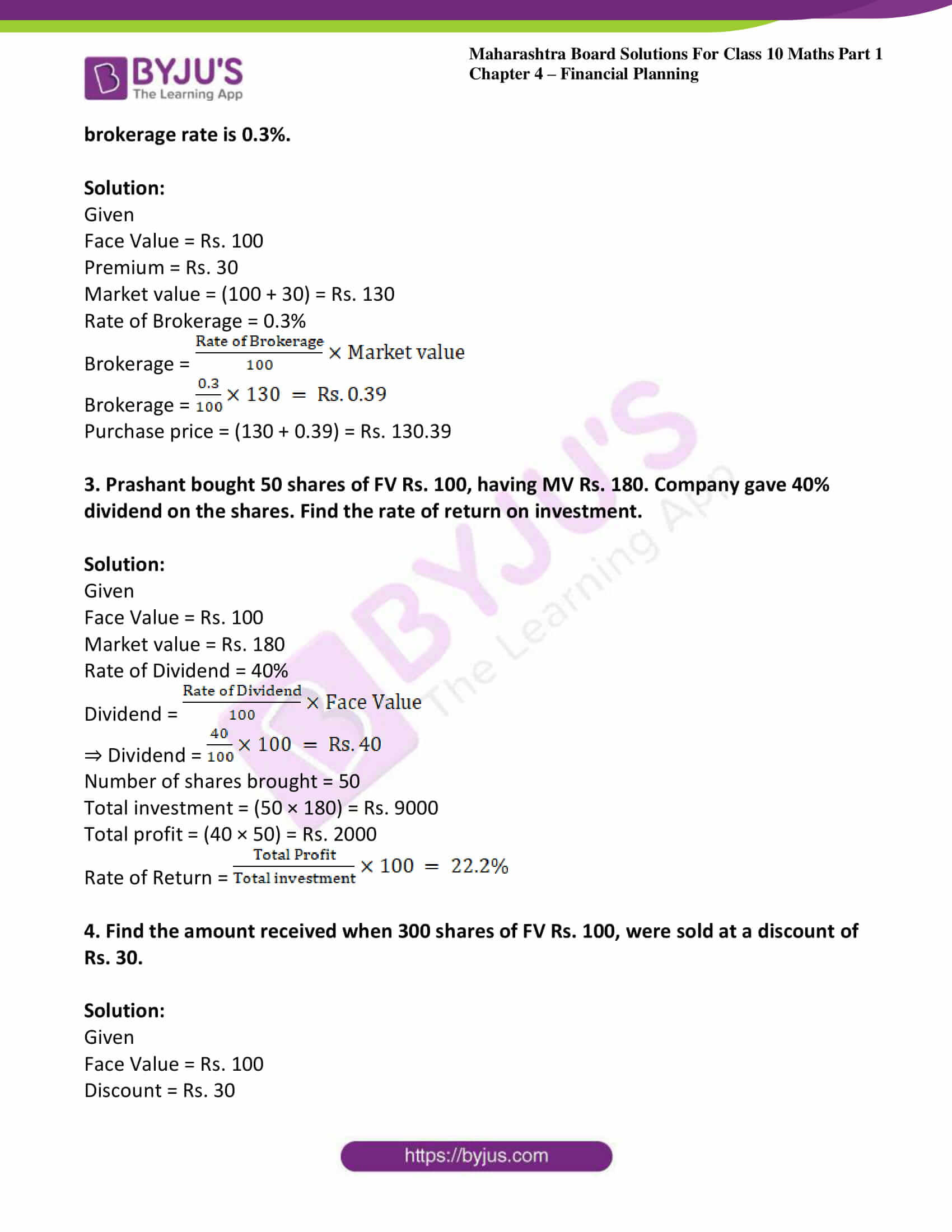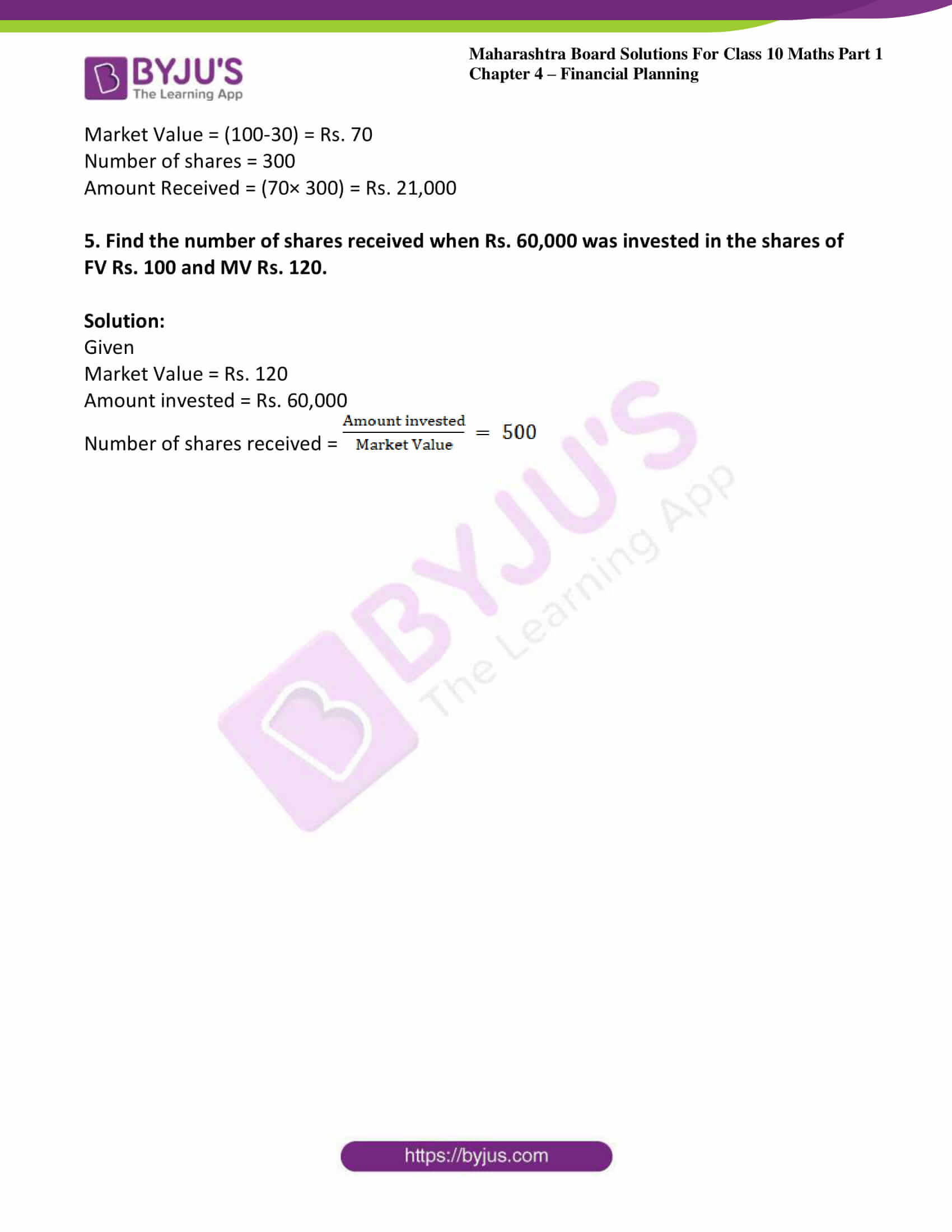### Access answers to Maths MSBSHSE Solutions For SSC Part 1 Chapter – Financial Planning

Practice set 4.1 Page no: 87

1. ‘Pawan Medical’ supplies medicines. On some medicines the rate of GST is 12%, then what is the rate of CGST and SGST?

Solution:

We know that CGST and SGST are components of GST.

CGST is always equal to SGST

That is GST = CGST + SGST

Let CGST and SGST be x.

Then GST = x + x

⇒ 12 = 2x

⇒ x = 6%

Hence CGST, SGST = 6%

2. On certain article if rate of CGST is 9% then what is the rate of SGST? and what is the rate of GST?

Solution:

We know that CGST and SGST are components of GST.

CGST is always equal to SGST.

∴ CGST = SGST = 9%

We know that GST = CGST + SGST.

∴ GST = 9% + 9% = 18%

3. ‘M/s. Real Paint’ sold 2 tins of lustre paint and taxable value of each tin is Rs. 2800. If the rate of GST is 28%, then find the amount of CGST and SGST charged in the tax invoice.

Solution:

Given rate of GST = 28%

The taxable value of 1 tin = Rs.2800

∴ Taxable value of 2 tins = Rs.5600

We know that CGST and SGST are components of GST.

CGST is always equal to SGST

i.e. GST = CGST + SGST

Let CGST and SGST be x.

Then GST = x + x

⇒ 28 = 2x

⇒ x = 14%

Hence CGST, SGST = 14%

We know that CGST =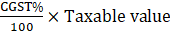⇒ CGST = 14/100 × 5600

= Rs.784

∵ CGST = SGST

∴ SGST = Rs.784

4. The taxable value of a wrist watch belt is Rs. 586. Rate of GST is 18%. Then what is price of the belt for the customer?

Solution:

Given rate of GST = 18%

Taxable value of wrist watch belt = Rs.586

We know that CGST and SGST are components of GST.

CGST is always equal to SGST

i.e. GST = CGST + SGST

Let CGST and SGST be x.

Then GST = x + x

⇒ 18 = 2x

⇒ x = 9%

Hence CGST, SGST = 9%

We know that CGST =⇒ CGST =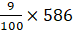= Rs.52.74

∵ CGST = SGST

∴ SGST = Rs.52.74

We know that price of a customer = Taxable value + CGST + SGST

∴ Price of belt for customer = 586 + 52.74 + 52.74

= 691.48

Practice set 4.2 Page no: 93

1. ‘Chetana Store’ paid total GST of Rs. 1,00,500 at the time of purchase and collected GST Rs. 1,22,500 at the time of sale during 1st of July 2017 to 31st July 2017. Find the GST payable by Chetana Stores.

Solution:

Given

Output Tax (tax collected at the time of sale) = Rs. 1,22,500

Input Tax (tax paid at the time of purchase) = Rs. 1,00,500

∴ Input Tax Credit, ITC = Rs. 1, 00, 500

We know that GST Payable = Output Tax – ITC

⇒ GST Payable by Chetana stores = 1, 22, 500 – 1, 00, 500

= Rs. 22, 000

2. Nazama is a proprietor of a firm, registered under GST. She has paid GST of Rs. 12,500 on purchase and collected Rs. 14,750 on sale. What is the amount of ITC to be claimed? What is the amount of GST payable?

Solution:

Given

Output Tax (tax collected at the time of sale) = Rs. 14,750

Input Tax (tax paid at the time of purchase) = Rs. 12,500

∴ Input Tax Credit, ITC = Rs. 12, 500

We know that GST Payable = Output Tax – ITC

⇒ GST Payable = 14, 750 – 12, 500

= Rs. 2, 250

3. Amir Enterprise purchased chocolate sauce bottles and paid GST of Rs. 3800. He sold those bottles to Akbari Bros. and collected GST of Rs. 4100. Mayank Food Corner purchased these bottles from Akabari Bros and paid GST of Rs. 4500. Find the amount of GST payable at every stage of trading and hence find payable CGST and SGST.

Solution:

Amir Enterprise:

Input GST = Rs.3800

Output GST = Rs. 4100

∴ GST Payable = Output Tax – ITC

= 4100 – 3800 = Rs. 300

We know that CGST and SGST are components of GST.

CGST is always equal to SGST

i.e. GST = CGST + SGST

Let CGST and SGST be x.

Then GST = x + x

⇒ 300 = 2x

⇒ x = 150

∴ CGST, SGST = Rs. 150

Akbari Bros:

Input GST = Rs. 4100

Output GST = Rs. 4500

∴ GST Payable = Output Tax – ITC

= 4500 – 4100 = Rs. 400

We know that CGST and SGST are components of GST.

CGST is always equal to SGST

i.e. GST = CGST + SGST

Let CGST and SGST be x.

Then GST = x + x

⇒ 400 = 2x

⇒ x = 200

∴ CGST, SGST = Rs. 200

Practice set 4.3 Page no: 102

1. Complete the following table by writing suitable numbers and words.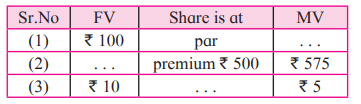Solution:

(1) Given that if MV = FV, then the share is at par.

Here, FV = Rs. 100

∴ MV = Rs. 100

(2) Given that if MV > FV, then the share is at premium.

∴ FV = MV – Premium

⇒ FV = 575 – 500 = Rs. 75

(3) Given that FV = Rs. 10 and MV = Rs.3

⇒ FV – MV = 10 – 5 = Rs. 5

We know that if MV < FV, then the share is at discount.

∴ The given share is at discount at Rs. 5.

2. Amol purchased 50 shares of Face Value Rs. 100 when the Market value of the share was Rs. 80. Company had given 20% dividend. Find the rate of return on investment.

Solution:

Given FV = Rs. 100; MV = Rs. 80; D = 20%

On investment of Rs. 80, Amol got Rs. 20.

Let rate of return be x%.

20/80 = x/ 100

¼ = x/100

x = 100/4

⇒ x = 25% (Rate of Return)

3. Joseph purchased following shares, Find his total investment.

Company A: 200 shares, FV = Rs. 2 Premium = Rs. 18.
Company B: 45 shares, MV = Rs. 500
Company C: 1 share, MV = Rs. 10,540.

Solution:

Company A:

We know that if MV > FV, then the share is at premium.

∴ MV = FV + Premium

⇒ MV = 2 + 18 = Rs.20

We know that investment = number of shares × MV

∴ Investment in company A = 200 × 20

= Rs. 4000

Company B:

∴ Investment in company B = 45 × 500

= Rs. 22, 500

Company C:

∴ Investment in company C = 1 × 10, 540

= Rs. 10, 540

Joseph has invested 4000 + 22, 500 + 10, 540 = Rs. 37, 040

Practice set 4.4 Page no: 109

1. Market value of a share is Rs. 200. If the brokerage rate is 0.3% then find the purchase value of the share.

Solution:

Given, MV = Rs. 200

Brokerage rate = 0.3%

We know that purchase value of a share = MV + Brokerage

∴ Purchase value of a share = 200 + 0.3% of 200

= 200 + 0.3/100 × 200

= 200 + 0.60

= Rs. 200.60

2. A share is sold for the market value of Rs. 1000. Brokerage is paid at the rate of 0.1%. What is the amount received after the sale?

Solution:

Given, MV = Rs. 1000

Brokerage rate = 0.1%

We know that the selling price for a share = MV – Brokerage rate

∴ Selling price for a share = 1000 – 0.1% of 1000

= 1000 – 1

= 999

3. Fill in the blanks given in the contract note of sale-purchase of shares.
(B – buy S – sell)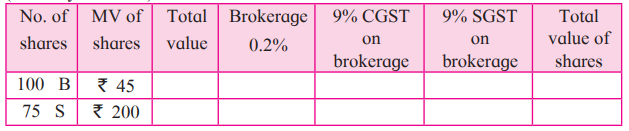Solution:

We know that Investment (total value) = Number of shares × MV

∴ Total value = 100 × 45

= Rs. 4500

We know that brokerage = Total Value × Brokerage rate

∴ Brokerage = 4500 × 0.2%

= Rs. 9

We know that CGST on brokerage = brokerage × CGST rate

∴ CGST on brokerage = 9 × 9%

= Rs. 0.81

We know that CGST value is equal to SGST value.

∴ SGST on brokerage = Rs. 0.81

We know that total value of shares = Total Value + Brokerage + CGST on brokerage + SGST on brokerage

⇒ Total value of shares = 4500 + 9 + 0.81 + 0.81

= Rs. 4510.62

S-Sell:

Total value = 200 × 75

= Rs. 15000

Brokerage = 15000 × 0.2%

= Rs. 30

CGST on brokerage = 30 × 9%

= Rs. 2.70 = SGST on brokerage

Total value of shares = 15000 + 30 + 2.70 + 2.70

= Rs. 14964.60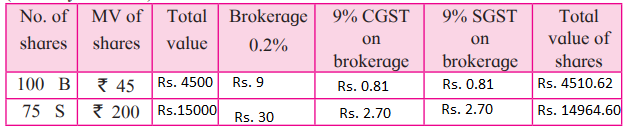Problem set 4a Page no: 109

1. Write the correct alternative for each of the following.
(1) Rate of GST on essential commodities is . . .
A. 5%
B. 12%
C. 0%
D. 18%

Solution:

C. 0%

Explanation:

According to Central board of Exercise and customs the rate of GST on essential commodities is 0%

(2) The tax levied by the central government for trading within state is . . .
A. IGST
B. CGST
C. SGST
D. UTGST

Solution:

B. CGST

Explanation:

The tax levied by the central government for trading is Central Goods and Services Tax.

(3) GST system was introduced in our country from . . .
A. 31st March 2017
B. 1st April 2017
C. 1st January 2017
D. 1st July 2017

Solution:

D. 1st July 2017

Explanation:

GST system was introduced from 1st July 2017

(4) The rate of GST on stainless steel utensils is 18%, then the rate of State GST is . . .
A. 18%
B. 9%
C. 36%
D. 0.9%

Solution:

B. 9%

Explanation:

According to Central board of Exercise and customs the rate of GST on stainless steels and utensils is 9%

(5) In the format of GSTIN there are . . . alpha-numerals.
A. 15
B. 10
C. 16
D. 9

Solution:

A. 15

Explanation:

GSTIN has 15 alpha numerals

(6) When a registered dealer sells goods to another registered dealer under GST, then this trading is termed as . . .
A. BB
B. B2B
C. BC
D. B2C

Solution:

B. B2B

Explanation:

The trading when a dealer sells goods to another registered dealer under GST is termed as B2B.

2. A dealer has given 10% discount on a showpiece of Rs. 25,000. GST of 28% was charged on the discounted price. Find the total amount shown in the tax invoice. What is the amount of CGST and SGST?

Solution: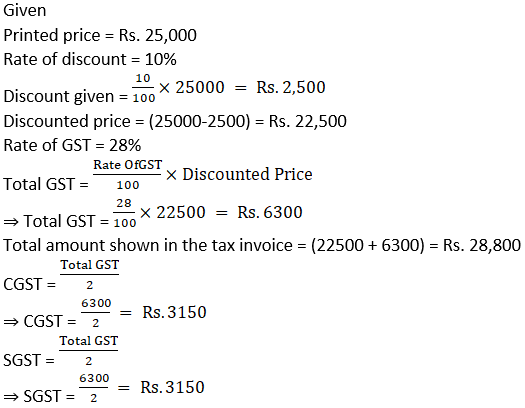3. A ready-made garment shopkeeper gives 5% discount on the dress of Rs. 1000 and charges 5% GST on the remaining amount, then what is the purchase price of the dress for the customer?

Solution: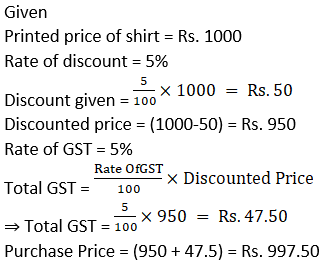4. A trader from Surat, Gujarat sold cotton clothes to a trader in Rajkot, Gujarat. The taxable value of cotton clothes is Rs. 2.5 lacs. What is the amount of GST at 5% paid by the trader in Rajkot?

Solution: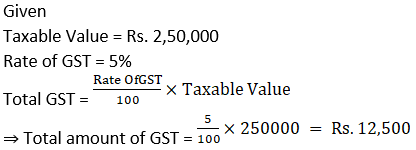5. Smt. Malhotra purchased solar panels for the taxable value of Rs. 85,000. She sold them for Rs. 90,000. The rate of GST is 5%. Find the ITC of Smt. Malhotra. What is the amount of GST payable by her?

Solution: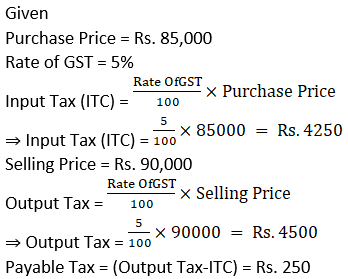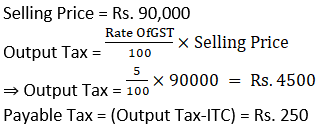Problem set 4b Page no: 111

1. Write the correct alternative for the following question.
(1) If the Face Value of a share is Rs. 100 and Market value is Rs. 75, then which of the following statements is correct?
A. The share is at premium of Rs. 175
B. The share is at discount of Rs. 25
C. The share is at premium of Rs. 25
D. The share is at discount of Rs. 75

Solution:

B. The share is at discount of Rs. 25

Explanation:

Market value = Rs. 75

Face Value = Rs. 100

If the market value is at a lower price than the face value then the share is in a discount.

Discount = (Face Value-Market value) = Rs. 25

(2) What is the amount of dividend received per share of face value Rs. 10 and dividend declared is 50%.
A. Rs. 50
B. Rs. 5
C. Rs. 500
D. Rs. 100

Solution:

B. Rs. 5

Explanation:

Rate of dividend = 50%

Dividend =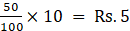(3) The NAV of a unit in mutual fund scheme is Rs. 10.65 then find the amount required to buy 500 such units.
A. 5325
B. 5235
C. 532500
D. 53250

Solution:

A. 5325

Explanation:

Net Asset Value = (N.A.V. of one unit × No. Of units) = Rs. 5325

(4) Rate of GST on brokerage is . . .
A. 5%
B. 12%
C. 18%
D. 28%

Solution:

C. 18%

Explanation:

Rate of GST on Brokerage is 18% according to Central board of Exercise and customs

(5) To find the cost of one share at the time of buying the amount of Brokerage and GST is to be . . . the MV of share.
B. subtracted from
C. Multiplied with
D. divided by

Solution:

Explanation:

The Brokerage and GST needs to be added to the Market Value of share at the time of buying.

2. Find the purchase price of a share of FV Rs. 100 if it is at premium of Rs. 30. The brokerage rate is 0.3%.

Solution:

Given

Face Value = Rs. 100

Market value = (100 + 30) = Rs. 130

Rate of Brokerage = 0.3%

Brokerage =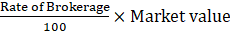Brokerage =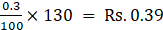Purchase price = (130 + 0.39) = Rs. 130.39

3. Prashant bought 50 shares of FV Rs. 100, having MV Rs. 180. Company gave 40% dividend on the shares. Find the rate of return on investment.

Solution:

Given

Face Value = Rs. 100

Market value = Rs. 180

Rate of Dividend = 40%

Dividend =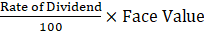⇒ Dividend =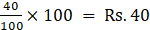Number of shares brought = 50

Total investment = (50 × 180) = Rs. 9000

Total profit = (40 × 50) = Rs. 2000

Rate of Return =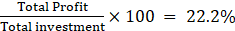4. Find the amount received when 300 shares of FV Rs. 100, were sold at a discount of Rs. 30.

Solution:

Given

Face Value = Rs. 100

Discount = Rs. 30

Market Value = (100-30) = Rs. 70

Number of shares = 300

Amount Received = (70× 300) = Rs. 21,000

5. Find the number of shares received when Rs. 60,000 was invested in the shares of FV Rs. 100 and MV Rs. 120.

Solution:

Given

Market Value = Rs. 120

Amount invested = Rs. 60,000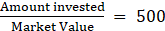## Frequently Asked Questions on Maharashtra State Board Solutions for Class 10 Maths Part 1 Chapter 4 Financial Planning

### Are these Maharashtra State Board Class 10 Maths Part 1 Chapter 4 Solutions useful to prepare for the exams?

Yes, these Maths Part 1 Chapter 4 Solutions are very helpful. Students are recommended to practise these solutions after revising the subjects because they set the basis for the questions that could get asked often in the board exams.